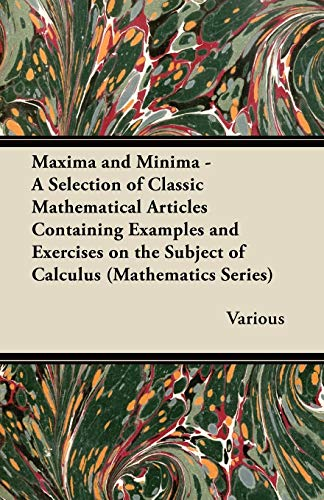• Free Shipping on all orders in Australia
• Over 7 million books in stock
• Proud to be B-Corp
• We aim to be carbon neutral by 2022
• Over 120,000 Trustpilot reviews
Item 1 of 0# Maxima and Minima - A Selection of Classic Mathematical Articles Containing Examples and Exercises on the Subject of Calculus (Mathematics Series) by Various

Condition - New
\$35.69
Only 2 left

## Maxima and Minima - A Selection of Classic Mathematical Articles Containing Examples and Exercises on the Subject of Calculus (Mathematics Series) Summary

### Maxima and Minima - A Selection of Classic Mathematical Articles Containing Examples and Exercises on the Subject of Calculus (Mathematics Series) by Various

Unfortunately we do not have a summary for this item at the moment

NLS9781447456858
9781447456858
1447456858
Maxima and Minima - A Selection of Classic Mathematical Articles Containing Examples and Exercises on the Subject of Calculus (Mathematics Series) by Various
New
Paperback